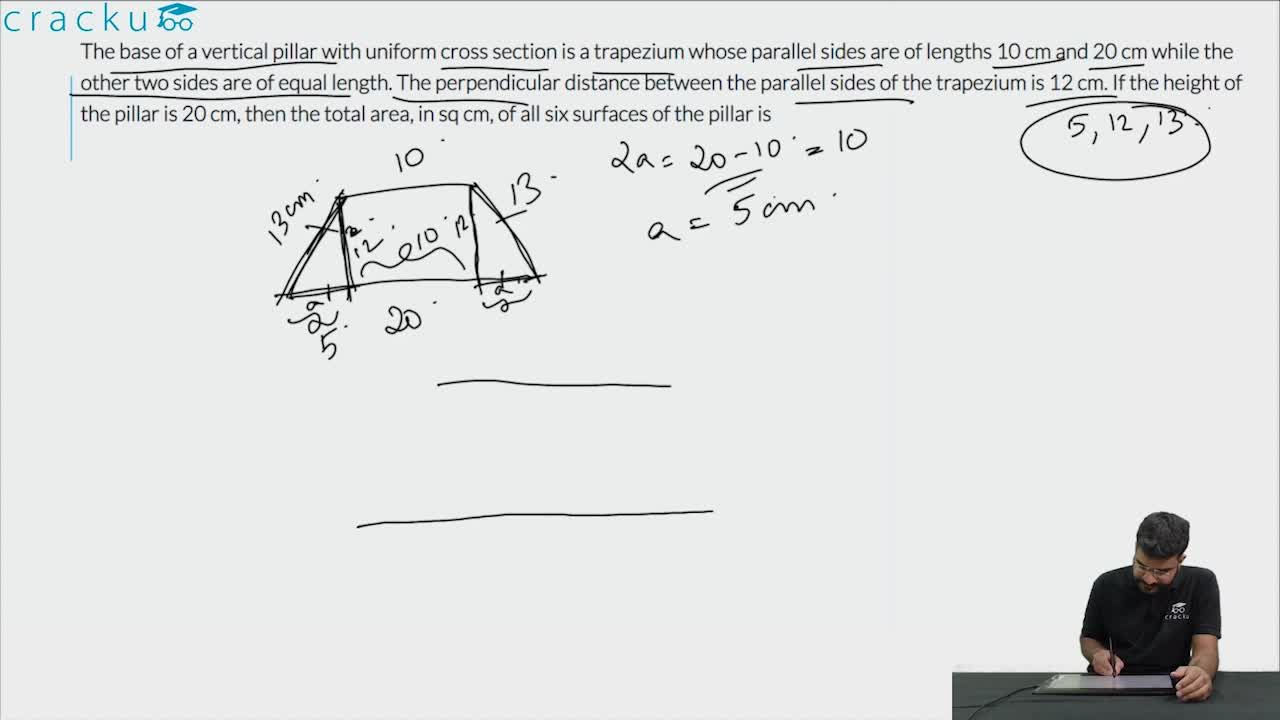Question 16

# The base of a vertical pillar with uniform cross section is a trapezium whose parallel sides are of lengths 10 cm and 20 cm while the other two sides are of equal length. The perpendicular distance between the parallel sides of the trapezium is 12 cm. If the height of the pillar is 20 cm, then the total area, in sq cm, of all six surfaces of the pillar is

Solution

See the diagram belowLength of side AD = $$\sqrt{12^2 + 5^2 } = 13$$
Area of the trapezium = 12 * (10 + 20)/2 = 180
Perimeter of the trapezium = 10 + 20 + 13 + 13 = 56
Area of the sides of the pillar = 56 * height  = 56 * 20 = 1120.
Total are of the pillar = 1120 + area of base + area of the top = 1120 + 180 + 180 = 1480

### View Video Solution